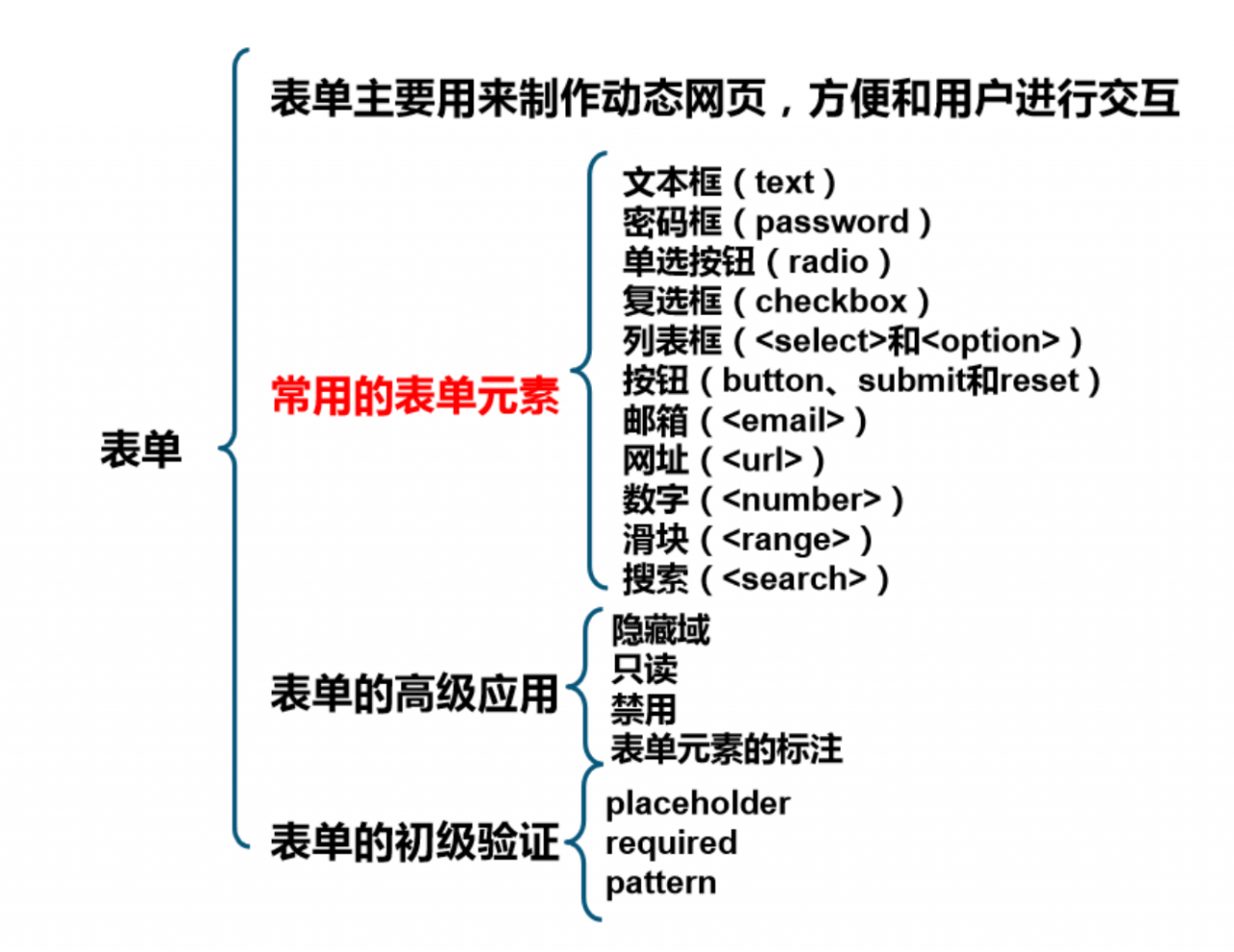# HTML 表单详解

1、表单语法

 1 2 3 4 5 6 7 8 9 10 11 `method: 规定如何发送表单数据常用值：get post` `在实际网页开发中通常采用post方式提交表单数据` `action: 表示向何处发送表单数据` `<``form` `method="post" action="result.html">` `    ``<``p``>名字：<``input` `name="name" type="text" > ` `    ``<``p``>密码：<``input` `name="pass" type="password" > ` `    ``<``p``>` `        ``<``input` `type="submit" name="Button" value="提交"/>` `        ``<``input` `type="reset" name="Reset" value="重填"/>` `    ``` ``

2、13个表单元素

1、文本框

 1 2 3 4 5 6 7 `` `<``input` `type="text" name="userName" value="用户名" size="30" maxlength="20"/>`

2、密码框

 1 2 3 4 5 `` `<``input` `type="password" name="pass" size="20"/>`

3、单选按钮

 1 2 3 4 5 6 7 `` `<``input` `name="gen" type="radio" value="男" checked />男` `<``input` `name="gen" type="radio" value="女" />女`

4、复选框

 1 2 3 4 5 6 7 8 `` `<``input` `type="checkbox" name="interest" value="sports"/>运动` `<``input` `type="checkbox" name="interest" value="talk" checked />聊天` `<``input` `type="checkbox" name="interest" value="play"/>玩游戏`

5、下拉列表框

 1 2 3 4 5 6 `` `` `<``select` `name="列表名称" size="行数">` `<``option` `value="选项的值" selected="selected">…` `<``option` `value="选项的值">…` ``

6、按钮

 1 2 3 4 5 6 7 8 `` `<``input` `type="reset" name="butReset" value="reset按钮">` `` `<``input` `type="submit" name="butSubmit" value="submit按钮">` `` `<``input` `type="button" name="butButton" value="button按钮"/>` `` `<``input` `type="image" src="images/login.gif" />`

7、多行文本域

 1 2 3 4 `textarea：多行文本域` `cols：显示的列数` `rows：显示的行数` `<``textarea` `name="showText" cols="x" rows="y">文本内容 `

8、文件域

 1 2 3 4 5 6 7 8 `enctype：表单编码属性` `<``form` `action="" method="post" enctype="multipart/form-data">` `<``p``>` `` `<``input` `type="file" name="files" />` `<``input` `type="submit" name="upload" value="上传" />` `` ``

9、邮箱

 1 `邮箱:<``input` `type="email" name="email"/>`

10、网址

 1 `请输入你的网址:<``input` `type="url" name="userUrl"/>`

11、数字

 1 2 3 4 `min：最小值` `max：最大值` `step：步长` `请输入数字:<``input` `type="number" name="num" min="0" max="100" step="10"/>`

12、滑块

type值为range即为滑块。

 1 `请输入数字:<``input` `type="range" name="range1" min="0" max="10" step="2"/>`

13、搜索框

type值为search即为搜索框。

 1 `请输入搜索的关键词:<``input` `type="search" name="sousuo"/>`

3、表单的高级应用

• 隐藏域

 1 `<``input` `type="hidden" value="666" name="userid">`
• 只读、禁用

W3C HTML5标准中，规定对于布尔类型的属性，属性值可以省略

 1 2 3 `只读和禁用不能单写readonly或disabled，必须写readonly＝”readonly”和disabled=“disabled”` `<``input` `name="name" type="text" value="张三" readonly>` `<``input` `type="submit" disabled value="保存" >`
• 表单元素的标注

 1 2 3 `` `<``label` `for="id">标注的文本` `<``input` `type="radio" name="gender" id="male"/>`

4、表单的初级验证1. 减轻服务器的压力。
2. 保证数据的可行性和安全性。

• placeholder

 1 `<``input` `type="search" name="sousuo" placeholder="请输入要搜索的关键字"/>`
• required

 1 `<``input` `type="text" name="username" required/>`
• pattern

 1 `<``input` `type="text" name="tel" required pattern="^1\d{9}" />`

5、小结